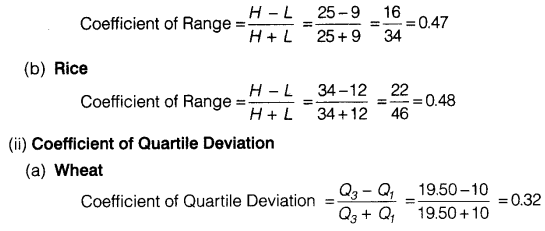• +91 9971497814
• info@interviewmaterial.com

# Chapter 6- Measures of Dispersion Interview Questions Answers

### Related Subjects

Question 1 :
A measure of dispersion is a good supplement to the central value in understanding a frequency distribution. Comment.

Dispersion is the extent to which values in a distribution differ from the avarage of the distribution. Knowledge of only average is insufficient as it does not reflect the quantum of variation in values.

Measures of dispersion enhance the understanding of a distribution considerably by providing information about how much the actual value of items in a series deviate from the central value, e.g., per capita income gives only the average income but a measure of dispersion can tell you about income inequalities, thereby improving the understanding of the relative living standards of different sections of the society. Through value of dispersion one can better understand the distribution.

Thus a measure of dispersion is a good supplement to the central value in understanding a frequency distribution.

Question 2 :
Which measure of dispersion is the best and how?

Standard Deviation is considered to be the best measure of dispersion and is therefore the most widely used measure of dispersion.

1. It is based on all values and thus provides information about the complete series. Because of this reason, a change in even one value affects the value of standard deviation.
2. It is independent of origin but not of scale.
3. It is us’eful in advanced statistical calculations like comparison of variability in two data sets.
4. It can be used in testing of hypothesis.
5. It is capable of further algebraic treatment.

Question 3 :
Some measures of dispersion depend upon the spread of values whereas some calculate the variation of values from a central value. Do you agree?

Yes, it is true that some measures of dispersion depend upon the spread of values, whereas some calculate the variation of values from the central value. Range and Quartile Deviation measure the dispersion by calculating the spread within which the value lie. Mean Deviation and Standard Deviation calculate the extent to which the values differ from the average or the central value.

Question 4 :
In town, 25% of the persons earned more than ₹ 45,000 whereas 75% earned more than 18,000. Calculate the absolute and relative values of dispersion.

25% of the persons earned more than ₹ 45,000. This implies that upper quartile Q3 = 45,000 75% earned more than 18,000. This implies that lower quartile Q1 =18,000
Absolute Measure of Dispersion = Q3 – Q1 = 45,000 – 18,000 = 27,000
Relative Measure of Dispersion
Co-efficient of Quartile Deviation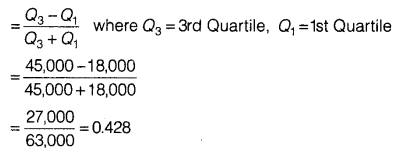Question 5 :
The yield of wheat and rice per acre for 10 districts of a state is as under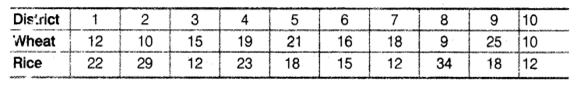Calculate for each crop,
(i) Range
(ii) QD
(v) Standard Deviation
(vi) Which crop has greater variation?
(vii) Compare the value of different measures for each crop.

(i) Range
(a) Wheat Highest value of distribution (H) = 25
Lowest value of distribution (L) = 9
Range = H – L = 25 – 9 = 16
(b) Rice Highest value of distribution (H) = 34
Lowest value of distribution (L)=12
Range = H – L = 34 – 12 = 22
(ii) Quartile Deviation
(a) Wheat Arranging the production of wheat in increasing order 9, 10, 10, 12, 15, 16, 18, 19, 21, 25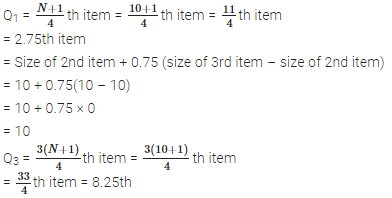= Size of 8th item + 0.25 (size of 9th item – size of 8th item)
= 19 + 0.25(21 – 19)
= 19 + 0.25 × 2
= 19 + 0.50 = 19.50
Quartile Deviation =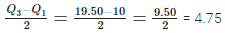(b) Rice Arranging the data of production of rice
12, 12, 12, 15, 18, 18, 22, 23, 29, 34 item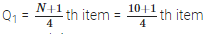= 2.75 th item
= Size of 2nd item + 0.75 (size of 3rd item – size of 2nd item)
= 12 + 0.75(12 – 12) = 12 + 0.75 × 0
= 12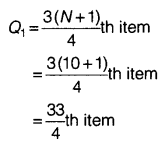= 8.25th item
= Size of 8th item + 0.25 (size of 9th item – size of 8th item)
= 23 + 0.25(29 – 23)
= 23 + 0.25 × 6
= 23 + 1.5
= 24.5
Quartile Deviation =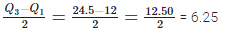(a) Wheat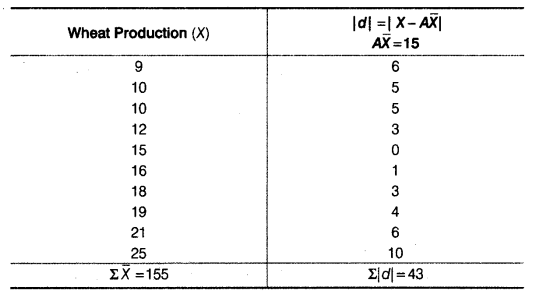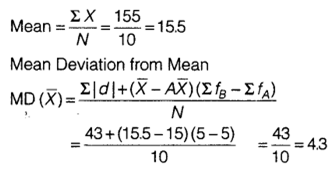(b) Rice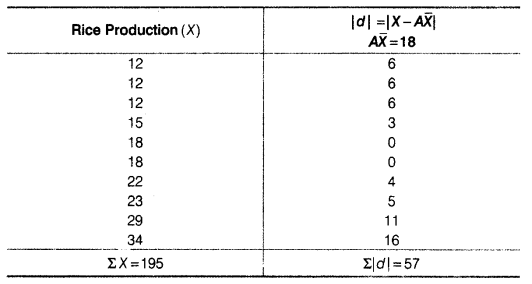(a) Wheat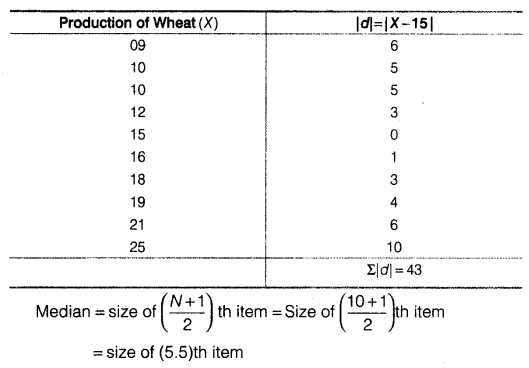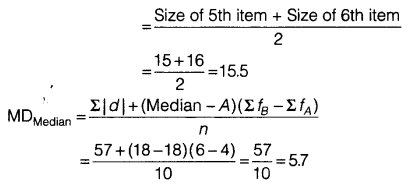(b) Rice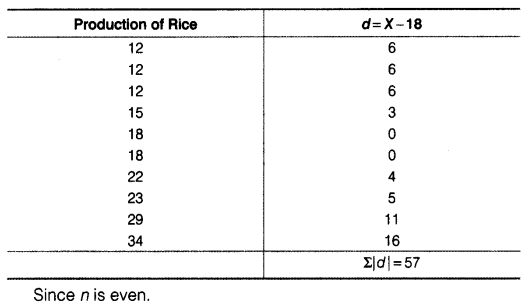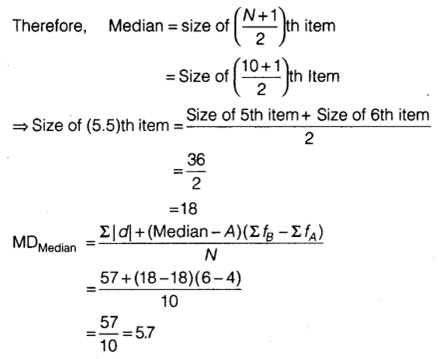(v) Standard Deviation
(a) Wheat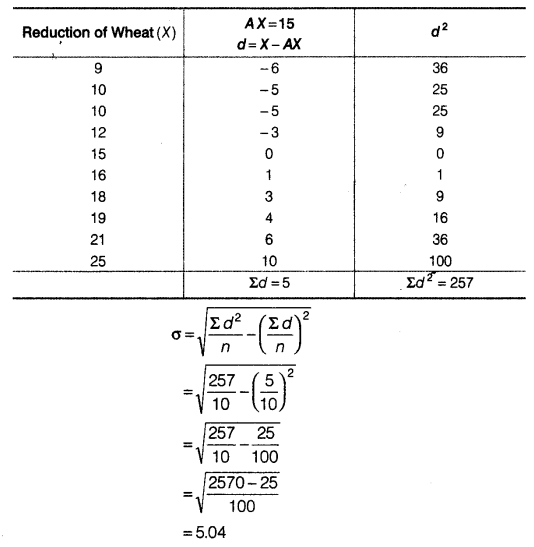(b) Rice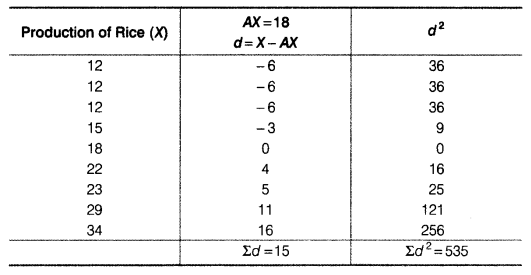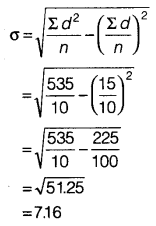(vi) Coefficient of Variation
(a) Wheat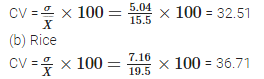Rice crop has greater variation as the coefficient of variation is higher for rice as compared to that of wheat.
(vii) Rice crop has higher Range, Quartile Deviation, Mean Deviation about Mean, Mean Deviation about Median, Standard Deviation and Coefficient of Variation.

Question 6 :
In the previous question, calculate the relative measures of variation and indicate the value which , in your opinion, is more reliable.